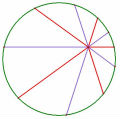# 函數具有性質 f(x-1)f(y-1)=f(xy-x-y+1)+2x-3y+3，求 f(2009)=?

## 函數具有性質 f(x-1)f(y-1)=f(xy-x-y+1)+2x-3y+3，求 f(2009)=?

$$f(1)f(2009)=f(2009)-6023$$ 及 $$f(2009)f(1)=f(2009)+4017$$，

^__^

左式＝$$f(x-1)f(y-1)=xy+y+x+1$$

右式＝$$f(xy-x-y+1)+2x-3y+3=xy-4y+x+6$$，兩者不必然會相等。

左式＝$$f(x-1)f(y-1)=4xy-2y-2x+1$$

右式＝$$f(xy-x-y+1)+2x-3y+3=-2xy-y+4x$$，兩者不必然會相等。

TOP

##### 引用:

weiye兄您好:

又f(y-1)*f(x-1)=f(yx-y-x+1)+2y-3x+3--------(ii)
(i)-(ii)  得 2x-3y+3=2y-3x+3  ,所以x=y
此時等號才成立...

[ 本帖最後由 Ellipse 於 2011-2-21 10:28 PM 編輯 ]

TOP

weiye兄您好:

TOP

TOP

^____^

TOP

##### 引用:

 老王 老王發短消息 加為好友 當前離線 8# 大 中 小 發表於 2011-3-21 18:09  只看該作者 個人淺見: 題目的寫法就應該是對任意x,y都正確，否則寫成x的式子就好， 也就是題目出錯了。 名豈文章著官應老病休飄飄何所似Essential isolated singularity UID261 帖子308 閱讀權限10 在線時間943 小時 註冊時間2009-5-14 最後登錄2014-3-17  查看詳細資料 TOP
﻿